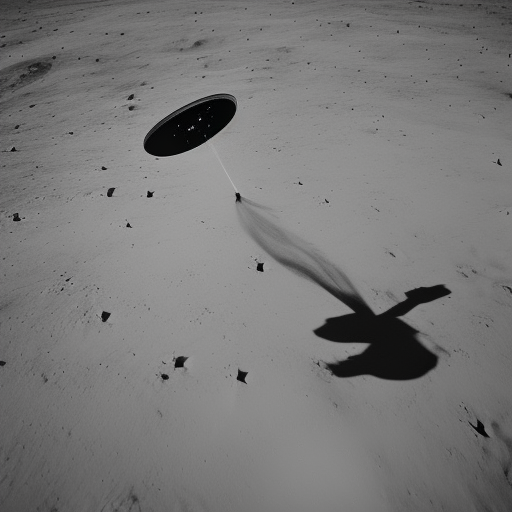David's Blog

# A Comprehensive Guide to Using Matplotlib in Python

By David Li on 2024-04-03T11:06:50.000Z# A Comprehensive Guide to Using Matplotlib in Python

Matplotlib is a powerful and versatile library in Python for creating static, interactive, and animated visualizations. It offers a wide variety of plots and chart types, making it the go-to library for many data analysts and scientists.

In this article, we will explore the fundamentals of Matplotlib, including installation, basic plots, customization, and advanced functionality.

## Installation

To install Matplotlib, simply use `pip`:

``pip install matplotlib``

Or, if you are using Anaconda, you can use `conda`:

``conda install matplotlib``

## Getting Started

Let’s begin by importing the necessary libraries:

``````import numpy as np
import matplotlib.pyplot as plt``````

We will use `numpy` to generate some sample data and `matplotlib.pyplot` to create our plots.

## Basic Plots

### Line Plot

A line plot is a basic type of plot that displays information as a series of data points connected by straight line segments. To create a line plot, use the `plot` function:

``````x = np.linspace(0, 10, 100)
y = np.sin(x)

plt.plot(x, y)
plt.show()``````### Scatter Plot

A scatter plot uses Cartesian coordinates to display values for two variables in a dataset. To create a scatter plot, use the `scatter` function:

``````x = np.random.rand(50)
y = np.random.rand(50)

plt.scatter(x, y)
plt.show()``````### Bar Plot

A bar plot represents categorical data with rectangular bars, where the length of the bars is proportional to the values they represent. To create a bar plot, use the `bar` function:

``````categories = ['A', 'B', 'C', 'D', 'E']
values = [3, 7, 2, 5, 8]

plt.bar(categories, values)
plt.show()``````### Histogram

A histogram is an approximate representation of the distribution of numerical data. To create a histogram, use the `hist` function:

``````data = np.random.randn(1000)

plt.hist(data, bins=30)
plt.show()``````## Customizing Plots

To add a title, x-axis label, and y-axis label, use the `title`, `xlabel`, and `ylabel` functions:

``````x = np.linspace(0, 10, 100)
y = np.sin(x)

plt.plot(x, y)
plt.title('Sine Wave')
plt.xlabel('x-axis')
plt.ylabel('y-axis')
plt.show()``````### Changing Colors and Line Styles

To change the color and line style of a plot, you can use the `color` and `linestyle` parameters:

``````x = np.linspace(0, 10, 100)
y1 = np.sin(x)
y2 = np.cos(x)

plt.plot(x, y1, color='blue', linestyle='-')
plt.plot(x, y2, color='green', linestyle='--')
plt.show()``````### Legend

To add a legend to your plot, use the `legend` function along with the `label` parameter in your plot:

``````x = np.linspace(0, 10, 100)
y1 = np.sin(x)
y2 = np.cos(x)

plt.plot(x, y1, label='sin(x)')
plt.plot(x, y2, label='cos(x)')
plt.legend()
plt.show()``````### Subplots

To create multiple plots in the same figure, use the `subplot` function:

``````x = np.linspace(0, 10, 100)
y1 = np.sin(x)
y2 = np.cos(x)

plt.subplot(2, 1, 1)
plt.plot(x, y1)
plt.title('sin(x)')

plt.subplot(2, 1, 2)
plt.plot(x, y2)
plt.title('cos(x)')

plt.tight_layout()
plt.show()``````### 3D Plots

To create 3D plots, you will need to import the `Axes3D` class from the `mpl_toolkits.mplot3d` module:

``````from mpl_toolkits.mplot3d import Axes3D

fig = plt.figure()

x = np.random.standard_normal(100)
y = np.random.standard_normal(100)
z = np.random.standard_normal(100)

ax.scatter(x, y, z)
ax.set_xlabel('X Label')
ax.set_ylabel('Y Label')
ax.set_zlabel('Z Label')

plt.show()``````### Animations

To create animations, you will need to import the `FuncAnimation` class from the `matplotlib.animation` module:

``````import matplotlib.animation as animation

fig, ax = plt.subplots()
x = np.linspace(0, 2 * np.pi, 100)
y = np.sin(x)
line, = ax.plot(x, y)

def update(frame):
y = np.sin(x + 0.1 * frame)
line.set_ydata(y)
return line,

ani = animation.FuncAnimation(fig, update, frames=range(100), interval=50, blit=True)

plt.show()``````

This code creates an animation of a sine wave, where the wave shifts to the right over time.

## Conclusion

In this article, we covered the basics of using Matplotlib in Python, including installation, creating basic plots, customizing plots, and exploring advanced functionality. Matplotlib is a powerful and flexible library that can help you create a wide variety of visualizations for your data analysis and presentation needs.

As you become more familiar with Matplotlib, you’ll find that it offers many more features and customization options than those covered in this article. For more information, consult the official Matplotlib documentation.

© Copyright 2023 by Astro Tech Blog. Built with ♥ by FriendlyUser. Last updated on 2023-05-29.